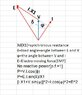# AC-SYN Generator

robax25
Nominal power PN= 3MVA
Nominal Voltage UN= 3000v (Y)
Line frequency f=50 Hz
Number of ole pairs= 2
Synchronous Reactance X1= 2.5 ohms

There is no reactive power and the generator can be assumed as lossless.

Formula and my solution,
I=PN/UN
I=3MVA/3000V
I=1000A

UN=XI +Up
Up= 3000v-2.5*1000A
UP=500v which is wrong

Load angel ? I don't know

•